### Home > MC2 > Chapter 9 > Lesson 9.3.3 > Problem9-114

9-114.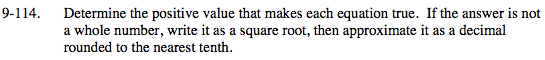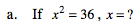What number multiplied by itself is 36?

${\it x} = \sqrt{36}$

x = 6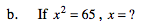Is 65 the square of any whole number?

$\sqrt{65} \text{ is approximately equal to 8.1.}$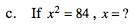Is 84 the square of any whole number?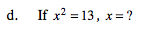Is 13 the square of any whole number?

x ≈ 3.6